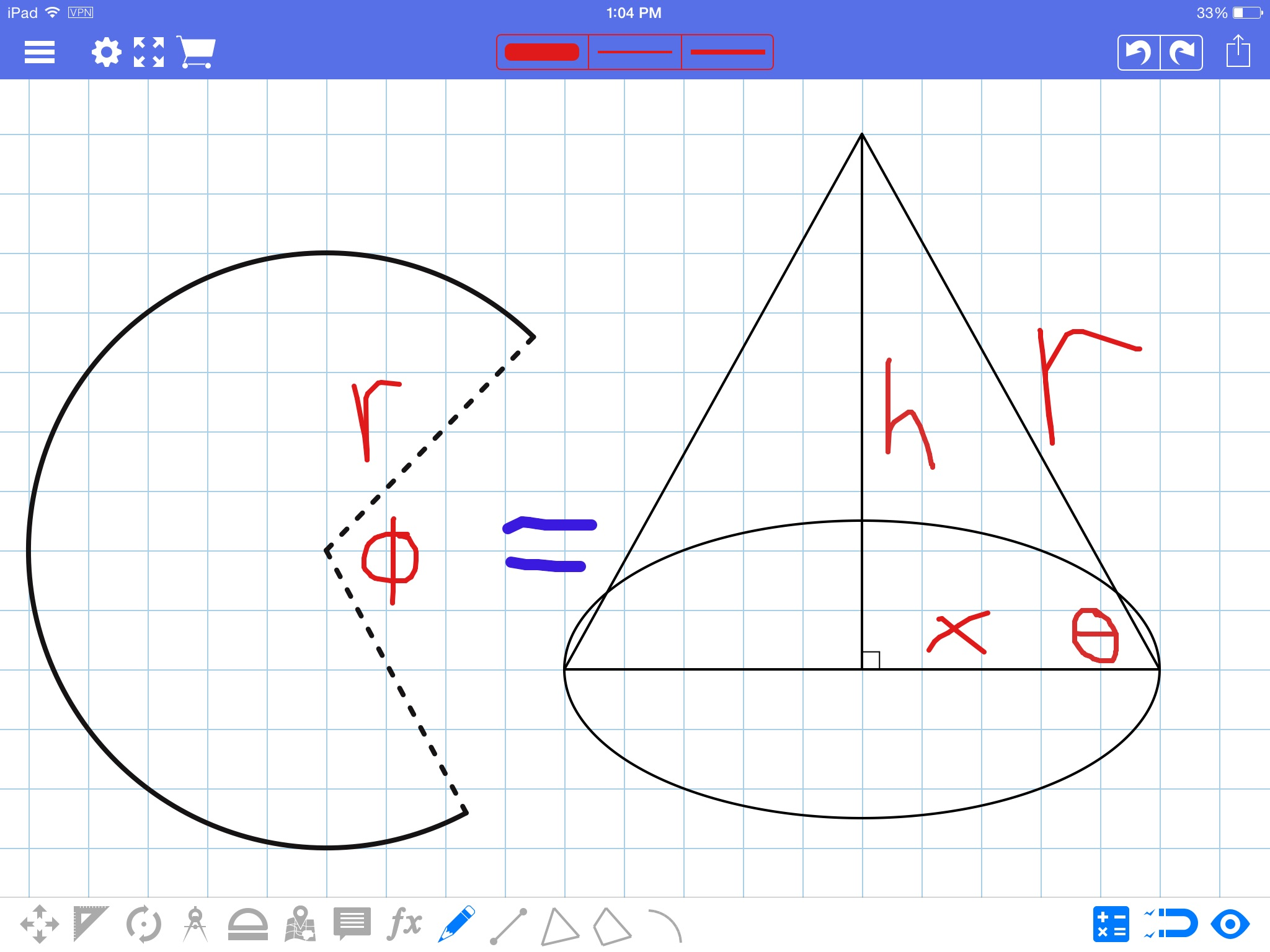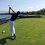# Generalized formulas: turning the circle into the coneRecently, I saw a problem on Facebook asking for the height of a cone created by removing a certain degree of a circle. So I decided to go above and beyond and derive a generalized form. IF YOU HAVE $ANY$ QUESTIONS, PLEASE ASK BECAUSE READINGS NOTHING WITHOUT UNDERSTANDING.

In this note, I will discuss a generalized formula for the height of a cone, angle of repose, radius of a cone's base, and the volume of a cone given the angle cut from the circle.

here is a list of the formulas:

$x=r\left(1-\dfrac{\phi}{360}\right)$

$\theta=\arccos \left(1-\dfrac{\phi}{360}\right)$

$h=\sqrt{\dfrac{\phi r^2}{180} \left(1-\dfrac{\phi}{720}\right)}$

$volume=\dfrac{\pi \times \sqrt{\dfrac{\phi r^2}{180} \left(1-\dfrac{\phi}{720}\right)} \times r^2\left(1-\dfrac{\phi}{360}\right)^2}{3}$

For those who aren't familiar with how to make a cone from a circle, click here to see how to make a cone from a circle Just start watching from where the video starts (it should start at3:45)

Refer to the above picture for reference to these terms. First, we begin by finding the radius of the cone. Because all circles are similar, the ratio of the cone base's circumference to the circle's circumference will be equivalent to the ratio of the cone's radius to the circles radius. $\dfrac{x}{r}=\dfrac{2\pi x}{2\pi r}$.

The cone base circumference=the circumference of the Pac-Man like circle (whats the mathematical term for this anyone?). Now to solve for the circumference of the cone's base in terms of r. The portion removed will be equivalent to $2\pi r(1-\dfrac{\phi}{360})$. Thus multiplying the ratio of the radii (or circumference since they're equivalent) by the radius of the Pac-Man, we get

$x=r\left(\dfrac{2\pi r(1-\dfrac{\phi}{360})}{2\pi r}\right)\Rightarrow r\left(1-\dfrac{\phi}{360}\right)$

Or in radians

$r\left(1-\dfrac{\phi}{2\pi}\right)$

If that was confusing, don't worry, everything after this is pretty straight forward.

Next, we will solve for $\theta$ aka, angle of repose. Because $\cos \theta =\dfrac{x}{r}$. We can solve for $\theta$ by using $\arccos \left(\dfrac{x}{r}\right)=\theta$. Thus we get

$\theta=\arccos \left(\dfrac{r\left(1-\dfrac{\phi}{360}\right)}{r}\right)\Rightarrow \arccos \left(1-\dfrac{\phi}{360}\right)$.

Or in radians

$\arccos \left(1-\dfrac{\phi}{2\pi}\right)$.

IF ANYONE HAS A GEOMETRIC FORMULA FOR THIS ANGLE PLEASE COMMENT IT.

Next, to solve for the height, we will simply use the Pythagorean theorem.

$h=\sqrt{r^2-x^2}$

$h=\sqrt{r^2-r^2\left(1-\dfrac{\phi}{360}\right)^2}$

$h=\sqrt{r^2-\left(r^2-\dfrac{2r^2 \phi}{360}+\dfrac{r^2\phi^2}{720\times 180}\right)}$

$h=\sqrt{\dfrac{r^2 \phi}{180}-\dfrac{r^2\phi^2}{720\times 180}}$

$h=\sqrt{\dfrac{r^2\phi }{180} \left(1-\dfrac{\phi}{720}\right)}$ or in radians $\sqrt{\dfrac{r^2\phi }{\pi} \left(1-\dfrac{\phi}{4\pi}\right)}$

Finally, solving for the volume is trivial (this isn't THAT useful because it's basically the normal process)

$volume=\frac{1}{3}hx^2=\dfrac{\pi \times \sqrt{\dfrac{\phi r^2}{180} \left(1-\dfrac{\phi}{720}\right)} \times r^2\left(1-\dfrac{\phi}{360}\right)^2}{3}$

Or in radians

$\frac{1}{3}hx^2=\dfrac{\pi \times \sqrt{\dfrac{\phi r^2}{\pi} \left(1-\dfrac{\phi}{4\pi}\right)} \times r^2\left(1-\dfrac{\phi}{2\pi}\right)^2}{3}$Note by Trevor Arashiro
6 years, 7 months ago

This discussion board is a place to discuss our Daily Challenges and the math and science related to those challenges. Explanations are more than just a solution — they should explain the steps and thinking strategies that you used to obtain the solution. Comments should further the discussion of math and science.

When posting on Brilliant:

• Use the emojis to react to an explanation, whether you're congratulating a job well done , or just really confused .
• Ask specific questions about the challenge or the steps in somebody's explanation. Well-posed questions can add a lot to the discussion, but posting "I don't understand!" doesn't help anyone.
• Try to contribute something new to the discussion, whether it is an extension, generalization or other idea related to the challenge.
• Stay on topic — we're all here to learn more about math and science, not to hear about your favorite get-rich-quick scheme or current world events.

MarkdownAppears as
*italics* or _italics_ italics
**bold** or __bold__ bold
- bulleted- list
• bulleted
• list
1. numbered2. list
1. numbered
2. list
Note: you must add a full line of space before and after lists for them to show up correctly
paragraph 1paragraph 2

paragraph 1

paragraph 2

[example link](https://brilliant.org)example link
> This is a quote
This is a quote
    # I indented these lines
# 4 spaces, and now they show
# up as a code block.

print "hello world"
# I indented these lines
# 4 spaces, and now they show
# up as a code block.

print "hello world"
MathAppears as
Remember to wrap math in $$ ... $$ or $ ... $ to ensure proper formatting.
2 \times 3 $2 \times 3$
2^{34} $2^{34}$
a_{i-1} $a_{i-1}$
\frac{2}{3} $\frac{2}{3}$
\sqrt{2} $\sqrt{2}$
\sum_{i=1}^3 $\sum_{i=1}^3$
\sin \theta $\sin \theta$
\boxed{123} $\boxed{123}$

## Comments

Sort by:

Top Newest

If you have any questions, please add them to this comment by hitting "reply". All questions are worth asking so feel free to ask anything.

- 6 years, 7 months ago

Log in to reply

×

Problem Loading...

Note Loading...

Set Loading...